Question

1. The Graduate Management Admission Test (GMAT) is a standardized exam used by many universities as part of the assessment for admission to graduate study in business. The average GMAT score is 547 (Magoosh website). Assume that GMAT scores are bell-shaped with a standard deviation of 100. What percentage of GMAT scores are 647 or higher

1.16% of GMAT scores are 647 or higher.

Step-by-step explanation:

Normal Probability Distribution:

Problems of normal distributions can be solved using the z-score formula.

In a set with mean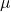and standard deviation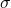, the z-score of a measure X is given by: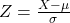The Z-score measures how many standard deviations the measure is from the mean. After finding the Z-score, we look at the z-score table and find the p-value associated with this z-score. This p-value is the probability that the value of the measure is smaller than X, that is, the percentile of X. Subtracting 1 by the p-value, we get the probability that the value of the measure is greater than X.

The average GMAT score is 547 (Magoosh website). Assume that GMAT scores are bell-shaped with a standard deviation of 100.

This means that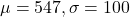What percentage of GMAT scores are 647 or higher?

The proportion is 1 subtracted by the p-value of Z when X = 647. So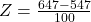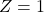has a p-value of 0.84.

1 – 0.84 = 0.16

0.16*100% = 16%

16% of GMAT scores are 647 or higher.